# Click-through Rate Prediction Based on Mobile Computing and Big Data Analysis

Click-through Rate Prediction Based on Mobile Computing and Big Data Analysis
China University of Geosciences Great Wall College, Baoding 071051, China

Hebei Finance University, Baoding 071051, China

Corresponding Author Email:
313660313@qq.com
Page:
313-319
|
DOI:
https://doi.org/10.18280/isi.240311
17 February 2018
|
Accepted:
6 May 2019
|
Published:
31 August 2019
| Citation

OPEN ACCESS

Abstract:

This paper designs a click-through rate (CTR) prediction model for ads based on mobile computing of the CTR logs of actual ads. The log preprocessing, feature extraction and model construction were conducted based on big data analysis. To preprocess to logs, an abnormal user detection method was developed based on power-law distribution. Then, the category features were extraction from user, context and ad. Next, the author proposed an evaluation model to predict the CRT of ads based on the extracted features. The experimental results verified the prediction accuracy of our model.

Keywords:

big data analysis, mobile computing, click-through rate (CTR), feature extraction, abnormal user

1. Introduction

The click-through rate (CTR) refers to the percentage of users viewing a webpage who click on a specific ad that appears on the page. The prediction of ad CTR is critical to the whole advertising industry, because it helps to prevent the display of low-quality, irrelevant ads on media platform. The user experience on the platform can be improved by presenting ads with the highest potential CTRs.

Online ads are displayed on digital media. The display and click events of an online ad are logged in real time, making it possible to measure the advertising effect. To optimize the ad targeting strategy, these logs have been frequently analyzed by big data technology. The existing ad targeting methods are either rule-based or model-based. The model-based approaches mainly examine the user features, context features or ad features of click events. In general, there are four steps of model-based ad targeting: the weighting of each feature with different big data analysis algorithms, the construction of an ad CTR prediction model, the estimation of the potential CRT of each ad, and the making of the optimal advertising decision.

Model-based ad targeting is one of the main applications of mobile computing [1-3], a novel technology combining the merits of mobile communication, Internet technology, distributed computing, etc. Mobile computing enables wireless data/resource sharing between smart information terminals like computers, and provides users with useful and accurate information anytime, anywhere. The most common method for model-based ad targeting is behavioral targeting. This method has been widely used by advertisers to customize the types of ads they receive based on the previous web browsing behavior of a web user. In behavioral targeting, the users are firstly divided into one or more groups; then, the similarity between an ad and each user group is computed; finally, the top user group is selected as the target group of the ad.

Many scholars have explored deep into behavioral targeting. For instance, Tu et al.  constructed portraits of users from their search records and browsing history, based on term frequency–inverse document frequency (TF-IDF). Sun et al.  set up a linear regression model to classify users based on their historical behavior. Allen et al.  modeled users by their interest changes in the short- and long-term. In addition, some scholars have analyzed the semantic information of user behavior, using latent semantic analysis models like latent semantic analysis , probability latent semantic analysis [8-9] and latent Dirichlet allocation . For example, Tang et al.  proposed a hybrid model that transforms the various input features by a decision tree, inputs the transformed features into a linear classifier, and then predicts the CTR of the target ad. Hur et al.  developed user portraits by time window, and used them to track the time-variation of user interests, thus acquiring  fresh big data on user interest.

This paper tackles the key issues of CTR prediction based on mobile computing and big data analysis, including data preprocessing, feature extraction and CTR estimation. Firstly, an abnormal user detection algorithm was designed based on power-law distribution, aiming to denoise the log data. Next, the ad CTR estimation features were extracted from different aspects. On this basis, a hybrid model was put forward to predict the ad CTR.

2. Data Analysis and Feature Extraction

The ad CTR prediction is a binary classification problem, which asks for mining user click behavior from numerous historical click logs.  To solve the problem, it is necessary to extract user and ad features of click events in an accurate manner. This calls for preprocessing of the original logs to eliminate the noises, which seriously affect the fitness of the CTR prediction model.

2.1 Data preprocessing based on mobile computing

In this research, the target dataset of click logs is obtained from the mobile Internet through mobile computing. There are 46 fields in the target dataset. The important ones are listed in Table 1 below.

Table 1. Important fields of the target dataset

 Field name Field meaning click the advertising is clicked or not timestamp click timestamp device device identity gender user’s gender age user’s age edu user’s education level interest user’s interest tag adid advertising’s ID city the city of the request os the type of user device OS cont_type the type of context
As shown in Figure 1, the daily log volume of ads ranges from 300,000 to 3 million.

## 1.png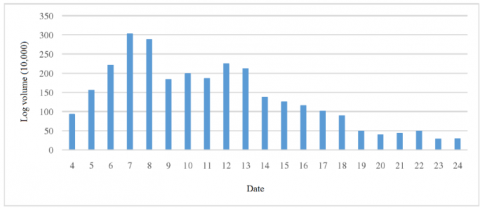Figure 1. Statistics on daily log volume

To eliminate the noises, the abnormal users in the dataset was detected through statistical analysis, considering the clear differences between abnormal and normal users in the statistical features of click behavior.

It is assumed that the relationship curve between the number of clicks on the ad and the number of users clicking on the ad obey the power-law distribution, a common distribution pattern for many natural and social data [13-15]. The power law distribution can be expressed as:

$y=a{{x}^{-p}}$   (1)

In this paper, each user is identified by the device field, and the total number of clicks field in the dataset is counted for each device. Then, the clicks field is sorted in descending order.

Table 2. The top ten users and total number of clicks

 Device field Clicks field 21342d24 63216 5ef4043f 47893 6ea35459 30197 b17f0be3 22260 3e987128 18346 6f86a101 17631 2eb2470b 15431 Fa23f736 15191 4ba12d27 13789 a72fe261 12917
The top ten items of the descending sequence are listed in Table 2, and the device field was encrypted.

Taking the total number of clicks as the reduction, the number of users corresponding to each clicks field was counted, and sorted in ascending order of clicks field. The top ten and bottom five items are displayed in Tables 3 and 4, respectively.

Table 3. The top ten users and total number of clicks

 Clicks Users 0 4231512 1 566478 2 117201 3 36855 4 17736 5 11238 6 6673 7 4112 8 2398 9 1778
Table 4. The bottom five users and total number of clicks
 Clicks Users 16761 1 22350 1 28021 1 46616 1 51099 1
The middle part was omitted, for the statistical results basically obeyed the long tail distribution. It can be seen that 4.15 million users never clicked on the ad, 569,000 users clicked on the ad only once, and only 1 user contributed an extremely high number of clicks. Then, the logarithmic relationship between the total number of clicks (x-axis) and the corresponding number of users (y-axis) was plotted (Figure 2).

## 2.png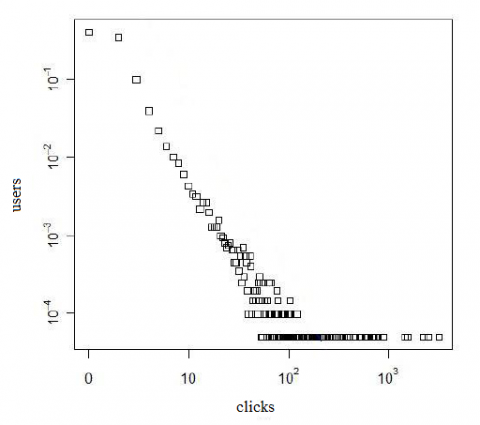Figure 2. The logarithmic relationship between the total number of clicks and that of users

As shown in Figure 2, the relationship curve is roughly a straight line, i.e. obeys power-law distribution, before the total number of clicks reaches 100; after that, the relationship curve is close to the horizontal line, that is, no longer obeys power-law distribution. Hence, 100 was selected as the threshold for the detection of abnormal users. The data of users which clicked more than 100 times were removed.

Figure 3 compares the data volume of normal users with that of abnormal users. It can be inferred that, out of the 27.16 million data, 23.38 million belong to normal users and about 3.78 million belong to abnormal users.

## 3.pngFigure 3. Data volume comparison between normal and abnormal users

Next, the CTRs of normal user dataset, abnormal user dataset and the original dataset were computed separately. The results are presented in Figure 4 below.

## 4.png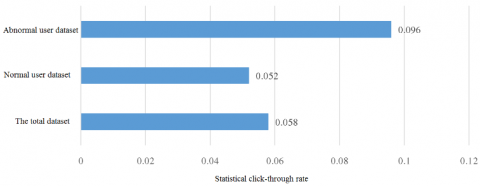Figure 4. The CTRs of different datasets

As shown in Figure 4, the CTR of the abnormal user dataset was much higher than that of the normal user dataset. Thus, the users identified as abnormal ones differed greatly from normal users, indicating the correctness of abnormal user detection.

2.2 Feature extraction

This subsection extracts features of the user features, the context and the ad from the historical data. Specifically, user features include age, gender and interest, ad features, the context features involve channel and time, and the ad feature refers to the keyword.

Before feature extraction, the pseudo-CTR was obtained from the offline dataset:

Pseudo-CTR $= ( \# \mathrm { C } ) / ( \# \mathrm { I } )$    (2)

where, #C is the number of clicks; #I is the total impressions (the number of times the ad is shown). However, the CTR directly computed from the historical logs often deviates from the actual data. In the case of a small total impressions, a slight variation in the number of clicks will bring a huge change to the CTR. To control the deviation of the CTR, a constant was introduced to the numerator and denominator of equation (2). In this way, the small total impressions will not cause a high inaccuracy, and the computed result will be close to the actual CTR. The revised CTR calculation formula is as follows:

Pseudo $- \mathrm { CTR } = ( \# \mathrm { C } + \alpha ) / ( \# \mathrm { I } + \alpha )$     (3)

where α is a smoothing parameter. The value of α should be determined based on the specific situation.

(1) Extraction of user features. Firstly, the historical CTRs of users in ten age groups, denoted as 0~10, were counted (Figure 5). The results show that the historical CTRs of different age groups varied from 0.03 to 0.07. Hence, age group was taken as a feature vector of ad CTR prediction.

## 5.pngFigure 5. Historical CTRs of all age groups

The historical CTRs of users with different genders were also counted. The results were normalized and plotted as a pie chart (Figure 6). Obviously, the historical CTR varied with user genders. Therefore, gender was also adopted as a feature vector of ad CTR prediction.

## 6.png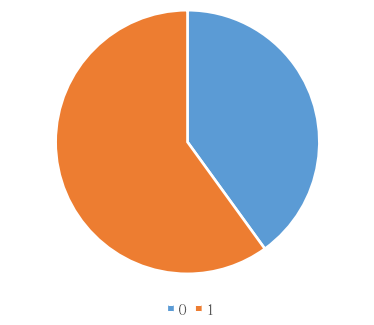Figure 6. Historical CTRs of both genders

In addition, the historical CTRs of users with different interests were counted and shown in Figure 7. If the users with an interest did not click on the ad, then the number of clicks corresponding to this interest was replaced with the mean number of clicks corresponding to all the other interests.

## 7.png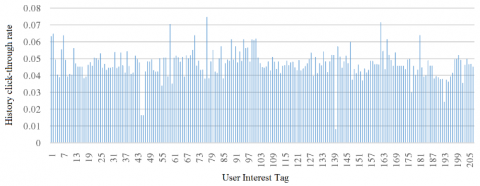Figure 7. Historical CTRs of varied interests

(2) Extraction of context features. The historical CTRs of the ad video in different channels were counted (Figure 8). Each channel stands for a category of ad videos.

## 8.pngFigure 8. Historical CTRs of different channels

## 9.png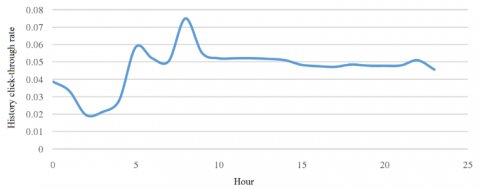Figure 9. History click-through rates at different times of the day

The historical CTRs of the ad appearing at different hours (0~23) were also counted (Figure 9). In the original dataset, there is a timestamp field in the format of “year-month-day hour: minute: second” (e.g. 2019-02-13 17:25:30). The results show that the CTR was constantly changing throughout the day. Thus, the hour and minute were both extracted, serving as two separate features for the prediction of ad CTR.

(3) Extraction of ad feature. Each ad in the original dataset has several keywords. The historical CTRs of each keyword were counted (Figure 10). It can be seen that the CTR varied with keywords. Hence, the keyword was taken as a feature vector to predict ad CTR.

## 10.pngFigure 10. Historical CTRs of different keywords

2.3 Statistical features

All the above features, including user features, context features and ad feature, are category features. This subsection sets up a statistical feature construction framework based on these category features. As shown in Figure 11, the framework includes following steps:

Step 1: All category features were divided into two feature sets S1 and S2, and a set of statistical indices I was set up, including mean, standard deviation, etc.

Step 2: The statistical feature was constructed sequentially from the first features in the two sets. For example, feature $S _ { 1 , i } ( 1 \leq i \leq m )$ of set S1 and feature $S _ { 2 , i } ( 1 \leq i \leq m )$ of set S2 were selected, forming a feature combination $\left\{ S _ { 1 , i } , S _ { 2 , i } \right\}$.

Step 3: Index Ii was selected from the set of statistical indices I, all data conforming to $\left\{ S _ { 1 , i } , S _ { 2 , i } \right\}$ were retrieved from the original dataset of the past 7 days, and then the value of index Ii was computed sequentially until reaching the last features of the two sets.

## 11.png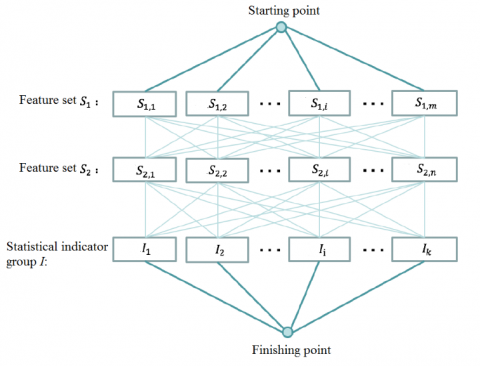Figure 11. The statistical feature construction framework

Let m be the number of features in set S1 and n be that in set S2. Then, a total of m×n×k statistical features can be constructed for each row of data, under the statistical feature construction framework. When the mean is selected as the statistical index, the resulting statistical feature is the historical CTR in the past 7 days CTR under the feature combination.

In this paper, the user, context and ad features are recombined with user ID, and then divided into two feature sets. One set contains 5 features and the other, 12 features. Using three statistical indices (i.e. mean (I1), sum (I2) and standard deviation (I3)), a total of 180 statistical features were constructed.

3. Hybrid Model for AD CTR Prediction

The most popular indicator of the CTR is eCPM, that is, the effective cost per thousand impressions (with M being the roman numeral for 1,000). The eCPM equals the product between the CTR μ and the effective cost per click ν. If ν is a constant, then eCPM is proportional to μ.

Each feature vector that contains the real-time information of click events was processed by an ad CTR prediction algorithm. A complete feature vector contains all three categories of information: user, context and ad. The ad CTR prediction algorithm learns and models the set of feature vectors, laying the basis for the CTR prediction model. During CTR prediction, the model converts the sample into a feature vector, and forecasts the value of the vector between zero and one. The forecasted value is the click probability. Our algorithm was constructed based on a hierarchical model for ad CTR prediction. As shown in Figure 12, the model learns the leaf weight by logistic regression.

For an input sample x, a binary sequence of falling points was obtained after the sample was processed by the integrating tree. Each falling point was marked as one, and each non-falling point, as zero. The sequence was regarded as a transformed feature, and subjected to logistic regression training to produce a logistic regression model.

## 12.png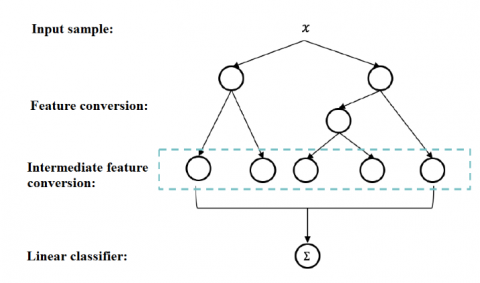Figure 12. The structure of the hierarchical model for ad CTR prediction

The hierarchical model, consisting of two layers (L1 and L2), was established after the click event dataset and feature matrix were derived from the original logs. The specific steps are summed up as Algorithm 1.

 Algorithm 1. Advertising click-through rate prediction algorithm Input: Advertising click training log L Output: Advertising click-through rate prediction hierarchical model 1: Clean data on the training log L, detect abnormal user on L, and obtain an abnormal user set U; 2: Filter log records related with U to form an advertising click data set T; 3: Extract and convert user features, advertising features, and context features on T to obtain a basic feature training matrix $\sum _ { \text {base } }$; 4: Extract statistical features on T and normalize them by column to get statistical feature training matrix $\sum _ { \text {stat } }$; 5: Splice the basic feature vector feature matrix $\sum _ { \text {base} }$ and the statistical feature training matrix $\sum _ { \text {stat } }$ by row to obtain a total training matrix $\sum _ { \text {T} }$; 6: Use $\sum _ { \text {T} }$ to train the integrated tree classifier to get the integrated tree classifier ML1; 7: Use ML1 to process on $\sum _ { \text {T} }$ to obtain the output matrix $\sum _ { \text {L1} }$ of L1; 8: Use $\sum _ { \text {L1} }$ to train the second layer classifier on $\sum _ { \text {T} }$ to obtain the model ML2; 9: Link ML1 and ML2 to get the full ad click-through rate prediction hierarchical model.

Next, a feature selection algorithm was developed based on Gini impurity and used to remove the redundant features of the statistical feature construction framework, which may otherwise affect the prediction accuracy or cause over-fitting. The feature selection algorithm was added to the above hierarchical model, forming the hybrid model for ad CTR prediction.

Gini impurity is a measure of data impurity in classification and regression tree (CART) . It means a result from a set is randomly applied to the expected error rate of a data item in the set. Gini impurity is used by the CART to construct a classified binary tree. The definition of Gini impurity is as follows:

$\operatorname { Gini } _ { \mathrm { C } } ( \mathrm { D } ) = 1 - \sum _ { 1 } ^ { \mathrm { m } } \mathrm { p } _ { \mathrm { i } } ^ { 2 }$    (4)

where, D is a dataset; C is a category in D; m is the number of C value; |Ci| is the number of samples in category C in D; |D| is the total number of samples in D; pi is the ratio of |Ci| to D:

$\mathrm { p } _ { \mathrm { i } } = \frac { \left| C _ { i } \right| } { D }$   (5)

Let R be the split attribute of C. Then, the split Gini impurity can be expressed as:

$\operatorname { Gin } _ { \mathrm { C } } ^ { \mathrm { TR } } ( \mathrm { D } ) = \frac { \left| \mathrm { D } _ { 1 } \right| } { | \mathrm { D } | } \operatorname { Gini } _ { \mathrm { C } } \left( \mathrm { D } _ { 1 } \right) + \frac { \left| \mathrm { D } _ { 2 } \right| } { | \mathrm { D } | } \operatorname { Gini } _ { \mathrm { C } } \left( \mathrm { D } _ { 2 } \right)$   (6)

where, D1 and D2 are the sub datasets after splitting. Thus, the increment of Gini impurity can be defined as:

$\Delta _ { \mathrm { R } } = \operatorname { Gini } _ { \mathrm { C } } ( \mathrm { D } ) - \operatorname { Gini } _ { \mathrm { C } } ^ { \mathrm { R } } ( \mathrm { D } )$       (7)

The largest attribute of R is the best split attribute.

Our feature selection algorithm (Algorithm 2) firstly fits the statistical features in turns, and then calculates the sum of the Gini impurity increments as the splitting attribute in the construction of integrated tree for each statistical feature. After that, the importance of each feature was determined according to the splitting attribute.

 Algorithm 2. Statistical feature selection algorithm Input: Statistical feature training matrix $\Sigma _ { \text {stat } }$ Output: Statistical feature training matrix $\Sigma _ { \text {selected} }$ after feature selection 1: Use $\Sigma _ { \text {stat } }$ training GBDT model, get the integrated tree structure SN of k trees, where tree list Τ={T1,T2,…,Ti,…,TK}; 2: The statistical feature set Μ={Μ1, Μ2,…,Μj,…,ΜN}, and Fj is the importance score of Mj, initialize Fj=0 when M=Mj: 2.1: Traverse Τ, for each split point of Mj, calculate the Gini impurity Gpar of its parent node; 2.2: Calculate the Gini impurity Gsplit after splitting according to the splitting of the Mj attribute; 2.3: ∆j=Gpar-Gsplit, Fj=Fj+∆j; 3: From 2. the statistical feature importance score list F={F1, F2,…,Fj,…,FN} is obtained, $\mathrm { F } _ { \text {total } } = \sum _ { \mathrm { j } - 1 } ^ { \mathrm { N } } \mathrm { F } _ { \mathrm { j } }$ 4: Normalize F according to $\mathrm { F } _ { \mathrm { j } } ^ { \prime } = \frac { \mathrm { F } _ { \mathrm { j } } } { \mathrm { F } _ { \text {total } } }$ and sort in descending order to get a list $\mathrm { F } ^ { \prime } = \left\{ \mathrm { F } _ { 1 } ^ { \prime } , \mathrm { F } _ { 2 } ^ { \prime } , \ldots , \mathrm { F } _ { \mathrm { j } } ^ { \prime } , \ldots , \mathrm { F } _ { \mathrm { N } } ^ { \prime } \right\}$ of statistical feature scores; 5: Initialize the feature list L=∅ and the accumulated variable δ=0, set the feature selection threshold λ (0≤λ≤1); 6: Traverse F' when $\mathrm { F } ^ { \prime } = \mathrm { F } _ { \mathrm { j } } ^ { \prime }$; 6.1: $\delta = \delta + F _ { j } ^ { \prime }$, if δ≤λ, the statistical feature corresponding to $\mathrm { F } _ { \mathrm { j } } ^ { \prime }$ is added to L; 6.2: Otherwise stop and break; 7: Obtain a statistical feature list L after feature selection; 8: Only retain the statistical features in L, and obtain the statistical feature training matrix $\Sigma _ { \text {selected } }$ after feature selection.

The filtering by Algorithm 2 eliminates the redundant statistical features, and improves the feature expression.

The selected feature vectors were imported to the hierarchical model of Algorithm1 to get the final hybrid model. The complete algorithm flow is shown as Algorithm 3.

 Algorithm 3. The construction algorithm of the hybrid model for ad CTR prediction Input: Training log L Output: Hybrid model for ad CTR prediction 1: Filter data in the training log L, detect abnormal users in L, and obtain a set of abnormal users U; 2: Filter the log records related to U to form a click event dataset T; 3: Extract and convert user, context and ad features in T, producing the basic feature training matrix $\Sigma _ { \text {base } }$; 4: Extract statistical features in T, and normalize them column by column, yielding the statistical feature training matrix $\Sigma _ { \text {stat } }$; 5: Use $\Sigma _ { \text {stat } }$ according to Algorithm 2 to obtain the statistical feature training matrix $\Sigma _ { \text {selected } }$ after feature selection; 6: Splice $\Sigma _ { \text {base } }$ and $\Sigma _ { \text {selected } }$ row by row to obtain the total training matrix $\Sigma _ { \mathrm { T } }$; 7: Use $\Sigma _ { \mathrm { T } }$ to train the integrated tree classifier to obtain the L1 integrated tree classifier ML1; 8: Use ML1 to process on $\Sigma _ { \mathrm { T } }$ to obtain the output matrix $\Sigma _ { \mathrm { L1} }$ of L1; 9: Use $\Sigma _ { \mathrm { L1 } }$ to train L2 on $\Sigma _ { \mathrm { T } }$ to obtain the model ML2; 10: Combined ML1 and ML2 into the hybrid model.
4. Experiment and Results Analysis

To verify its effectiveness, our hybrid model for ad CTR prediction was compared with traditional ad CTR prediction algorithms through experiments. The experimental dataset is the preprocessed dataset of click event logs. The prediction results were verified by sliding window. With a 7-day time-span for feature statistics and an 8-day long window, the first 7 days in the window were used as the training set, and the 8th day was taken as the test set. The window slid three times from July 17th, forming three sets of test data. The area under the curve (AUC) was selected to evaluate the test results. The mean AUC of the three window datasets was adopted as the evaluation criterion.

The prediction by our model was compared with that by several traditional ad CTR prediction algorithms. The mean results of the slides on July 20th, 21st and 22nd are displayed in Figure 13. It is clear that our model outperformed the other algorithms.

## 13.pngFigure 13. The mean results of our model and the other algorithms

In machine learning, there are two types of generalization errors: bias and variance. The former reflects the deviation of the predicted value from the actual value, that is, the fitting ability of the prediction model. The latter describes the variation in the training set of the same size, which changes the learning performance of the model and measures the data disturbance. Hence, the tradeoff between bias and variance is critical to the training of machine learning models. The XGBoost is a boosting algorithm that iteratively learns the residual of the previous iteration, and thus improves the model fitness. This algorithm can minimize the deviation if the variance is not too large, i.e. the base classifier has a small variance. Compared with this algorithm, our model tends to have a shallow and integrated tree.

During the experiment, the depth of the tree was set to 3, and the number of trees was adjusted constantly to converge to the optimal result. The initial number of trees was set to 50. This number was increased at the step size of 50 until reaching 280. The prediction results of our model and XGBoost are contrasted in Figure 14.

## 14.png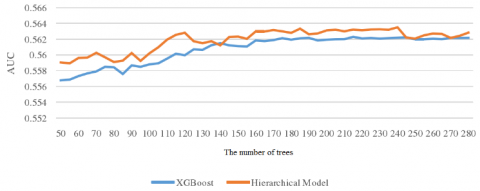Figure 14. The prediction results of our model and XGBoost

As shown in Figure 14, as the number of trees gradually increased from 50 to 280, our model and XGBoost achieved comparable results, and both converged to the optimal solution. Our model achieved the optimal result when the number of trees reached 240, with the depth of each tree being 3. Next, the number of trees was fixed to 240, and the depth of each tree was increased from 2 to 10. The change in the AUC is shown in Figure 15.

## 15.png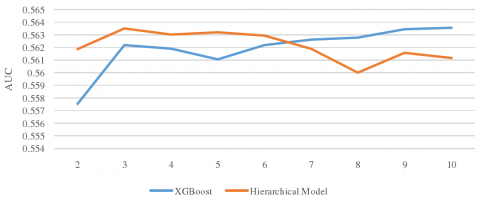Figure 15. Change of the AUC with tree depths

Obviously, our model achieved the best result at the tree depth of 3, showing a low complexity. With the growth in the number of trees, the trend of training time on L1 in our model is presented in Figure 16.

## 16.pngFigure 16. The trend of training time on L1

It can be seen from Figure 16 that the training time of L1 basically increased with the number of trees, averaging at 8.983 min.

5. Conclusions

This paper puts forward a hybrid model for ad CTR prediction based on based on mobile computing and big data analysis. Firstly, the integrated tree model was employed to convert the input features. Then, the converted features were inputted into a linear model for training, and output the structure of the hierarchical model. Next, a feature selection algorithm was added to the hierarchical model, forming the hybrid model. The experimental results show that our model achieved desirable results, thanks to the optimization of the important parameters.

Acknowledgment

This work is supported by Hebei Province University Smart Finance Application Technology R&D Center Project (XGJ2018008); Hebei Province Innovation Capacity Improvement Plan Project (18450136)This work is supported by Hebei Province University Smart Finance Application Technology R&D Center Project (XGJ2018008); Hebei Province Innovation Capacity Improvement Plan Project (18450136)

References

 Perera, C., Zaslavsky, A., Christen P., Georgakopoulos, D. (2014). Context aware computing for the internet of things: A survey. IEEE Communications Surveys & Tutorials, 16(1): 414-454. https://doi.org/10.1109/SURV.2013.042313.00197

 Sun, G., Bin, S. (2018). A new opinion leaders detecting algorithm in multi-relationship online social networks. Multimedia Tools and Applications, 77(4): 4295-4307.

 Wang, Q., Huang, K., Li, S., Yu, W. (2017). Adaptive modeling for large-scale advertisers optimization. Big Data Analytics, 2(1): 8-17. https://doi.org/10.1186/s41044-017-0024-6

 Tu, S., Huang, M. (2016). Mining microblog user interests based on textrank with TF-IDF factor. Journal of China Universities of Posts & Telecommunications, 23(5): 40-46. https://doi.org/10.1016/S1005-8885(16)60056-0

 Sun, G, Bin, S. (2017). Router-level internet topology evolution model based on multi-subnet composited complex network model. Journal of Internet Technology, 18(6): 1275-1283.

 Allen, S.M., Chorley, M.J., Colombo, G.B., Jaho, E., Karaliopoulos, M., Stavrakakis, I., Whitaker, R.M. (2014). Exploiting user interest similarity and social links for micro-blog forwarding in mobile opportunistic networks. Pervasive and Mobile Computing, 11: 106-131. https://doi.org/10.1016/j.pmcj.2011.12.003

 Gandon, F. (2018). A survey of the first 20 years of research on semantic web and linked data, Ingénierie des Systèmes d’Information, 23(3-4): 11-56. https://doi.org/10.3166/ISI.23.3-4.11-56

 Yan, M., Zhang, X., Yang, D., Xu, L., Kymer, J.D. (2016). A component recommender for bug reports using discriminative probability latent semantic analysis. Information & Software Technology, 73(C): 37-51. http://dx.doi.org/10.1016/j.infsof.2016.01.005

 Mezher, R., Omar, N. (2016). A hybrid method of syntactic feature and latent semantic analysis for automatic arabic essay scoring. Journal of Applied Sciences, 16(5): 209-215. http://dx.doi.org/10.3923/jas.2016.209.215

 Tuarob, S., Pouchard, L., Mitra, P., Giles, C.L. (2015). A generalized topic modeling approach for automatic document annotation. International Journal on Digital Libraries, 16(2): 111-128. https://doi.org/10.1007/s00799-015-0146-2

 Tang, J., Chang, Y., Liu, H. (2014). Mining social media with social theories. ACM SIGKDD Explorations Newsletter, 15(2): 20-29. http://dx.doi.org/10.1145/2641190.2641195

 Hur, W.M., Moon, T.W., Jung, Y.S. (2015). Customer response to employee emotional labor: the structural relationship between emotional labor, job satisfaction, and customer satisfaction. Journal of Services Marketing, 29(1): 71-80. http://dx.doi.org/10.1108/JSM-07-2013-0161

 Sun, G., Bin, S. (2017). Router-level internet topology evolution model based on multi-subnet composited complex network model. Journal of Internet Technology, 18(6): 1275-1283. http://dx.doi.org/10.6138/JIT.2017.18.6.20140617

 Zhao, K., Musolesi, M., Hui, P., Rao, W., Tarkoma, S. (2015). Explaining the power-law distribution of human mobility through transportation modality decomposition. Scientific Reports, 5: 9136. https://doi.org/10.1038/srep09136

 Liu, P., Xia, H. (2015). Structure and evolution of co-authorship network in an interdisciplinary research field. Scientometrics, 103(1): 101-134. https://doi.org/10.1007/s11192-014-1525-y